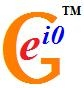# User blogs

Tag search results for: "riemann zeta function"Fractal Geography of the Riemann Zeta Function (by Chris King)

The quadratic Mandelbrot set has been referred to as the most complex and beautiful object in mathematics and the Riemann Zeta function takes the prize for the most complicated and enigmatic function. Here we elucidate the spectrum of Mandelbrot and Julia sets of Zeta, to unearth the geography of its chaotic and fractal diversities, combining these two extremes into one intrepid journey into the deepest abyss of complex function space.

Part I of this article includes: Introduction; A Bridge over Turbulent Waters; Chasing the Critical Points and their Parameter Planes; and A: The Additive World - 1: Far East - the Asymptotically-Critical Plateau; 2: Real Critical Points, from Miniscule to Vast; and (3) Shang-ri-La – The Unreal Criticals.

Part II of this article includes: B: The Multiplicative Universe; and Appendix: Fractal Geography of Eta, Xi and Dirichlet L-functions; and Introduction for Non-mathematicians.

· Oct 16 '12 ·Experimental Observations on the Uncomputability of the Riemann Hypothesis: Part I & II (by Chris King): Abstract: This paper seeks to explore whether the Riemann hypothesis falls into a class of putatively unprovable mathematical conjectures, which arise as a result of unpredictable irregularity. It also seeks to provide an experimental basis to discover some of the mathematical enigmas surrounding these conjectures, by providing Matlab and C programs which the reader can use to explore and better understand these systems (see appendix 6 in Part II).

Part I of this article includes: Introduction; The Riemann hypothesis and the Zeta Function; and The Quantum Chaos Connection. http://prespacetime.com/index.php/pst/article/view/355

Part II of this article includes: Julia and Mandelbrot sets of the Riemann Zeta Function; The Syracuse Shibboleth; and Appendices: 1. Moments of Zeta; 2. Zeta Bernoulli Numbers; 3. Twin Prime Distribution; 4. Other Dirichlet series for zeta; 5. Lanczos approximation to Gamma; 6. Mac XCode Viewer and Matlab Toolbox for Figures; and 7. A Formula for Depicting the Derivative of Zeta. http://prespacetime.com/index.php/pst/article/view/356

Fractal Geography of the Riemann Zeta Function: Part I & II (by Chris King): Abstract: The quadratic Mandelbrot set has been referred to as the most complex and beautiful object in mathematics and the Riemann Zeta function takes the prize for the most complicated and enigmatic function. Here we elucidate the spectrum of Mandelbrot and Julia sets of Zeta, to unearth the geography of its chaotic and fractal diversities, combining these two extremes into one intrepid journey into the deepest abyss of complex function space.

Part I of this article includes: Introduction; A Bridge over Turbulent Waters; Chasing the Critical Points and their Parameter Planes; and A: The Additive World - 1: Far East - the Asymptotically-Critical Plateau; 2: Real Critical Points, from Miniscule to Vast; and (3) Shang-ri-La – The Unreal Criticals. http://prespacetime.com/index.php/pst/article/view/357

Part II of this article includes: B: The Multiplicative Universe; and Appendix: Fractal Geography of Eta, Xi and Dirichlet L-functions; and Introduction for Non-mathematicians. http://prespacetime.com/index.php/pst/article/view/358

A Dynamical Key to the Riemann Hypothesis: Part I & II (by Chris King): Abstract: We investigate a dynamical basis for the Riemann hypothesis (RH) that the non-trivial zeros of the Riemann zeta function lie on the critical line x = ½. In the process we graphically explore, in as rich a way as possible, the diversity of zeta and L-functions, to look for examples at the boundary between those with zeros on the critical line and otherwise. The approach provides a dynamical basis for why the various forms of zeta and L-function have their non-trivial zeros on the critical line. It suggests RH is an additional unprovable postulate of the number system, similar to the axiom of choice, arising from the asymptotic behavior of the primes.

Part I of this article includes: Introduction; The Impossible Coincidence; Primes and Mediants - Equivalents of RH; A Mode-Locking View of Dirichlet L-functions and their Counterexamples; Widening the Horizon to other types of Zeta and L-Function; L-functions of Elliptic Curves; and Modular and Automorphic Forms. http://prespacetime.com/index.php/pst/article/view/359

Part II of this Article includes: Functions with Functional Equations but no Euler Product; A Central Showcase: Modular Forms Meeting Elliptic Functions; Seeking Examples with Product Formulae; Dynamically Manipulating the Non-trivial Zeros in and out of the ‘Forbidden Zone’; Finding Coefficient Paths with On-Critical Zeros; Conclusion; Appendix 1: Mediants and Mode-Locking; Appendix 2: Finite Fields and Square Roots of -1; Appendix 3: Derivation of Davenport Heilbronn; Appendix 4: A Comparison of Computational Methods; and Appendix 5: Useful Sage and PARI-GP Commands. http://prespacetime.com/index.php/pst/article/view/360

· Mar 19 '12 ·